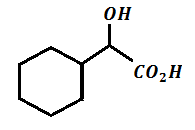(P)     ; Product (P) of the reaction is :

(65% yield)

(a)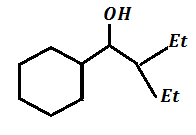(b)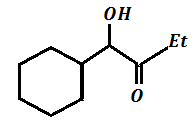(c)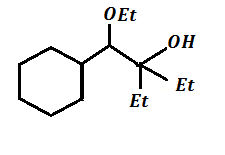(d)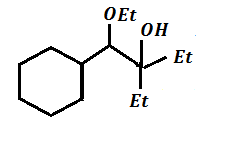Concept Questions :-

Preparation & properties of Aldehydes and Ketones
High Yielding Test Series + Question Bank - NEET 2020

Difficulty Level: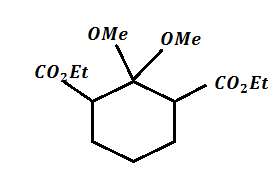$\underset{∆}{\overset{{\mathrm{H}}_{3}\mathrm{O}}{\to }}$ (A) , Product (A) obtained is :

(a)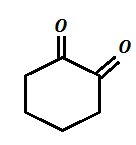(b)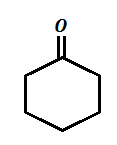(c)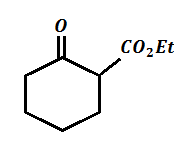(d)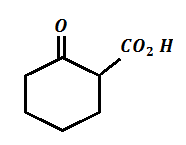Concept Questions :-

Acid Derivatives, Preparation and Properties
High Yielding Test Series + Question Bank - NEET 2020

Difficulty Level:

Which of the following acid on heating gives geometrical isomers as a product ?

(a)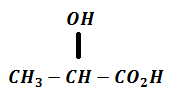(b)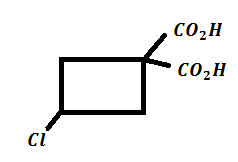(c)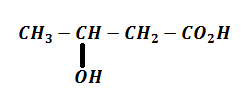(d) All of these

Concept Questions :-

Carboxylic Acids
High Yielding Test Series + Question Bank - NEET 2020

Difficulty Level: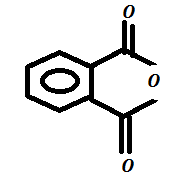$\stackrel{\mathrm{MeOH}}{\to }$A$\stackrel{{\mathrm{PCl}}_{3}}{\to }$B$\stackrel{{\mathrm{MeNH}}_{2}}{\to }$C ; Product (C) of the reaction is :

(a)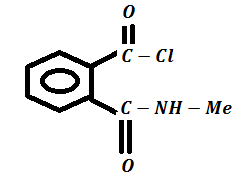(b)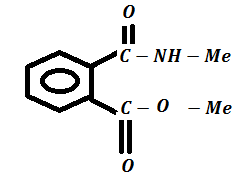(c)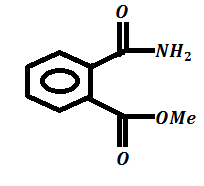(d)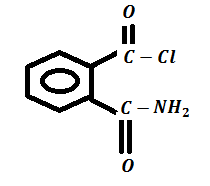High Yielding Test Series + Question Bank - NEET 2020

Difficulty Level: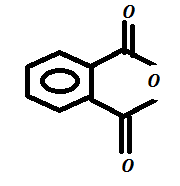$\stackrel{{\mathrm{LiAlH}}_{4}}{\to }$A$\stackrel{\mathrm{PCC}}{\to }$B$\stackrel{{\mathrm{NH}}_{2}-{\mathrm{NH}}_{2}}{\to }$C , Product (C) is :

(a)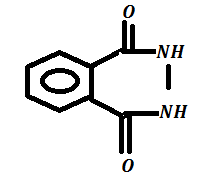(b)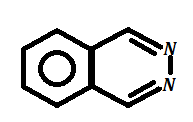(c)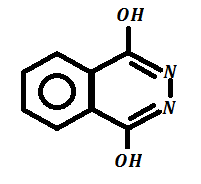(d)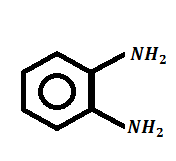Concept Questions :-

Acid Derivatives, Preparation and Properties
High Yielding Test Series + Question Bank - NEET 2020

Difficulty Level: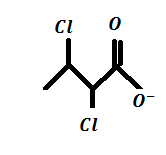$\stackrel{-{\mathrm{CO}}_{2}}{\to }$A$\stackrel{\mathrm{HCl}}{\to }$BC. Product (C) is :

(a)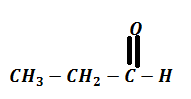(b)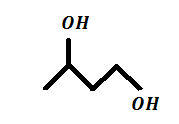(c)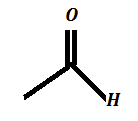(d)Concept Questions :-

Acid Derivatives, Preparation and Properties
High Yielding Test Series + Question Bank - NEET 2020

Difficulty Level: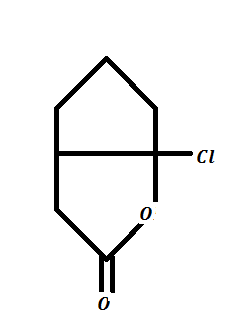+$\mathrm{R}-{\mathrm{CH}}_{2}-{\mathrm{NH}}_{2}$$\underset{\mathrm{THF}}{\overset{{\mathrm{K}}_{2}{\mathrm{CO}}_{3}}{\to }}$ (A)

(71%)

In above reaction identify major product (A) of the reaction:

(a)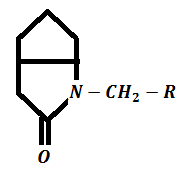(b)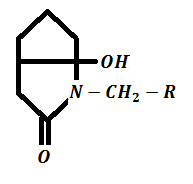(c)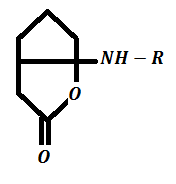(d)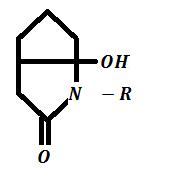Concept Questions :-

Preparation & properties of Aldehydes and Ketones
High Yielding Test Series + Question Bank - NEET 2020

Difficulty Level: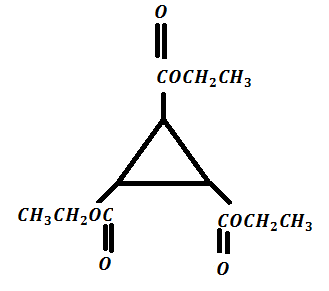$\stackrel{\mathrm{KOH}}{\to }$ A  $\stackrel{{\mathrm{H}}_{3}{\mathrm{O}}^{+}}{\to }$ B  $\stackrel{{\mathrm{PCl}}_{5}}{\to }$ C  $\stackrel{{\mathrm{LiAlH}}_{4}}{\to }$  D  $\stackrel{{\mathrm{H}}^{+}/∆}{\to }$(X)

Product (X) is :

(a)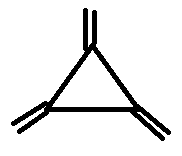(b)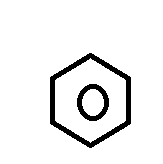(c)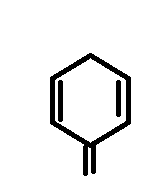(d)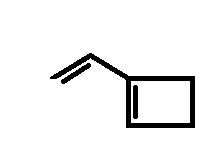Concept Questions :-

Acid Derivatives, Preparation and Properties
High Yielding Test Series + Question Bank - NEET 2020

Difficulty Level:

A key step in the hydrolysis of acetamide in aqueous acid proceeds by nucleophilic addition of :

(a) ${\mathrm{H}}_{3}{\mathrm{O}}^{+}$ to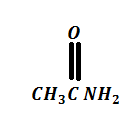(b)  ${\mathrm{H}}_{2}\mathrm{O}$ to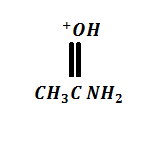(c) ${\mathrm{H}}_{3}{\mathrm{O}}^{+}$ to(d) ${\mathrm{HO}}^{-}$ toConcept Questions :-

Acid Derivatives, Preparation and Properties
High Yielding Test Series + Question Bank - NEET 2020

Difficulty Level:

All but one of the following compounds react with aniline to give acetanilide. Which one does not?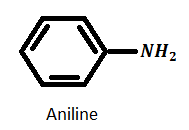$\to$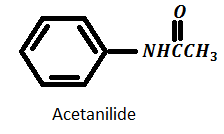(a)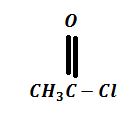(b)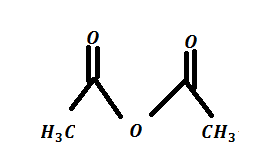(c)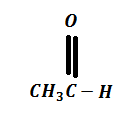(d)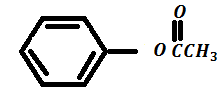Concept Questions :-

Acid Derivatives, Preparation and Properties